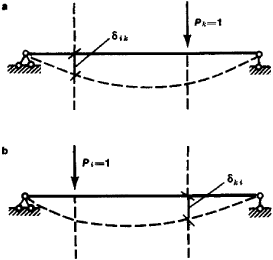Relative Motion, Principle of

Relative Motion, Principle of

(Maxwell’s theory), states that for a linearly deformed body the displacement 8ki of a point of application of a single force Pk of the first state (see Figure 1, a) in the direction of its action caused by any other single force P1 of the second state (see Figure 1, b) is equal to the displacement δik of the point of application of the force Pi in the line of action of single force Pk—that is, δik = δkiFigure 1. Displacements (deflections) of a simple beam under the influence of single forces: (a) first state, (b) second state

The principle of relative motion, first formulated by the English physicist J. Maxwell, is a special case of the principle of relative work; it is widely used in strength of materials and in structural mechanics in the calculation of elastic systems.

L. V. KASAB’IAN

Site: Follow: Share:
Open / Close